• graphics.h的头文件，C++编写的模拟流体运动
• matlab计算流体力学编程代码 之前本项目的是由C++实现的，代码实在混乱异常,极不利于维护。 现自己边学习，边使用python重新编写robocup 3d simulaiton 项目代码，并进行机器学习代码的编写（代码和书籍参考机器学习...
• 基于最近的数学进展，BhT 提供了一系列 M 文件，用于设计、模拟和分析关节体在流体中的运动。 更广泛地说，BhT 还允许轻松执行关于流体中固体运动的任何类型的数值实验（所谓的流固耦合系统的模拟）。 生物流体动力...
• potential flowhttp://simulations.narod.ru/This is simulation of liquid motion around object using potential flow method.The velocity field is represented as external velocity plus influence from objec...
potential flowhttp://simulations.narod.ru/This is simulation of liquid motion around object using potential flow method.The velocity field is represented as external velocity plus influence from object that considered as electric field in some electrostatic task.  potential flow means Laplace operator of potential is 0 that is electrostatic task. The electrostatic task is solved as set of point charge that charges need to find from condition for velocity to be tangent on object surface. All charges is on object surface or inside.It is possible to draw object. Spline interpolation used.Run main.mSee how it works in video:http://www.youtube.com/watch?v=VGngHjK_fm0
展开全文• 基于Matlab的Stokesian和Brownian动力学代码，用于模拟流体中的胶体运动 目的 计算在流体中扩散的胶体粒子的轨迹。 该代码可以： 考虑使用Rotne-Prager校正在斯托克斯近似下的粒子之间的流体动力相互作用。 考虑作用...
• CFDTool是基于FEATool Multiphysics™设计的，专门用于简单的流体动力学和热传导模拟仿真。该工具箱的主要特点包括： 完全独立可运行的CFD MATLAB仿真工具箱； 完全集成且易于使用的图形用户界面GUI； 一维...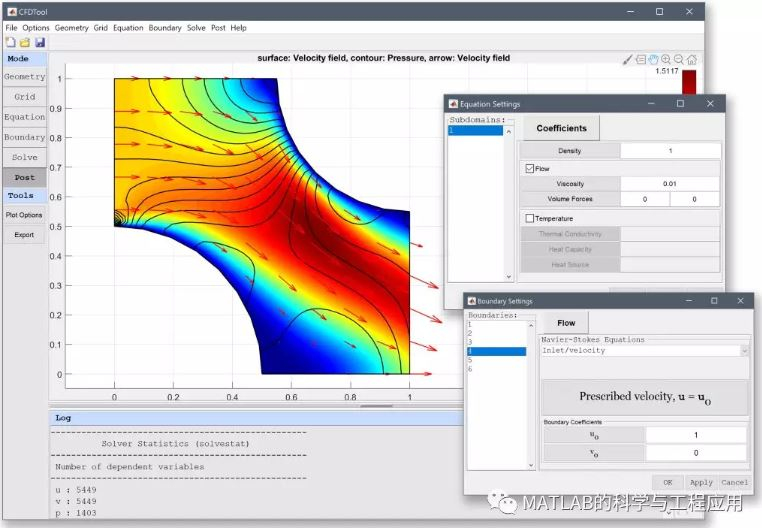CFDTool是基于FEATool Multiphysics™设计的，专门用于简单的流体动力学和热传导模拟仿真。该工具箱的主要特点包括：

完全独立可运行的CFD MATLAB仿真工具箱；


完全集成且易于使用的图形用户界面GUI；


一维、二维和轴对称圆柱坐标系的建模与仿真（包含旋流/非零方位角速度）；


内置几何学和CAD工具（支持平面STL CAD文件导入）；


自动网格生成（支持FEniCS、Gmsh、GiD、GMV和三角形网格格式的导入）；


不可压缩层流流动与热传导的预定义方程和边界条件；


定常和时间依赖的解算器；


边界和子域积分的后处理与可视化。


该工具箱可运行于Windows、Mac和Linux操作系统，要求MATLAB版本为R2009b及其以上。本工具箱的建模过程分为以下六个步骤：

几何：定义几何模型；


网格：将几何形状细分成适合计算的较小单元；


反应式：设定材料参数和系数的规格；


边界：边界条件指定模型如何与周围环境（几何之外）相互作用；


求解：模型问题的求解与仿真；


后处理：可视化与数据后处理。


工具箱下载地址：
http://page2.dfpan.com/fs/1lc1j2921729c16f614/
更多精彩文章请关注微信号：展开全文• Binous 和 RJ Phillips 使用 FORTRAN 77 程序发现的结果一致，动态模拟 FENE 哑铃粘弹性悬浮液中的一个和两个粒子沉降，非牛顿流体力学杂志，卷。 83, pp 93-130, 1999。有关控制方程和非牛顿流体的详细信息可以在...
• Stokesian Dynamics 是由 Brady 和 Bossis 在 80 年代开发的一种方法，可模拟低雷诺数下流体动力学相互作用球体的 3D 运动。 该程序最初由 Ron Phillips 教授于 1989 年在麻省理工学院攻读博士学位期间编写。该代码...
• *重力*拖*电梯*推力*浮力*大量流体运动，例如风*惯性力，例如离心力和科里奥利力在大多数入门物理学和动力学课程中，重力是唯一考虑的力（这相当于假设运动是在真空中进行的）。 在这里，我们将考虑现实而准确的...
• matlab开发-模拟动力学。不同工具的一阶线性系统仿真
• 开发工作集中在粘性流体中自由运动的数值模拟。 目的是模拟粘性流体中小物体的非常慢的运动。 我们通过寻找适用于现场变量的数值解决方案，开发了人体在粘性流体中的沉浸动力学模拟。 我们开发了用于垂直和球形圆柱...
• Stokesian Dynamics 是由 Brady 和 Bossis 在 80 年代开发的一种方法，可模拟低雷诺数下流体动力学相互作用球体的 3D 运动。 目前的贡献计算了牛顿流体的简单和振荡剪切流中流体动力学相互作用球体的轨迹。 作为...
• 流体模拟插件Jawset TurbulenceFD C4D v1.0 1437 for Cinema 4D R15-R20 TFD插件 中英双语版 含转VDB工具 含R20_GPU破译补丁(Win/Mac)注意：此破译版除R20之外只能使用CPU进行模拟Jawset TurbulenceFD C4D v1.0 1437...
流体模拟插件Jawset TurbulenceFD C4D v1.0 1437 for Cinema 4D R15-R20 TFD插件 中英双语版 含转VDB工具 含R20_GPU破译补丁(Win/Mac)注意：此破译版除R20之外只能使用CPU进行模拟Jawset TurbulenceFD C4D v1.0 1437 (简写为TFD插件)是一款C4D流体水墨烟雾特效插件，TurbulenceFD模拟管道实现了一个基于不可压缩Navier Stokes 方程的分析解算器。这意味着它使用一个立体像素网格，描述体积烟和火，解决了述流体运动的网格方程。每个立体像素TurbulenceFD计算流体的速度以及一些渠道来描述属性,如温度、烟密度、数量的燃料等等。这个模拟过程产生每一帧的体元网格,使用缓存的磁盘上的体积渲染器。Jawset TurbulenceFD V1.0 Rev 1437版本支持Cinema 4d R20。附件下载文件名称：TurbulenceFD C4D v1.0 1437更新日期：2018-12-23作者信息：资源来源于网络提示：下载后请检查MD5值，欢迎捐赠本站以及广告合作！
展开全文• 我们知道真实的液体是不可压缩的，但我们在计算机中离散的计算流体运动，在一定的时间步长内，用标准的SPH方法求解，在在粒子聚集处容易发生挤压，造成压缩。有两种常用的方法模拟不可压缩性：1.在WCSPH(弱可压缩SPH...
SPH（光滑粒子流体动力学）流体模拟实现五：PCISPH

我们知道真实的液体是不可压缩的，但我们在计算机中离散的计算流体运动，在一定的时间步长内，用标准的SPH方法求解，在在粒子聚集处容易发生挤压，造成压缩。有两种常用的方法模拟不可压缩性：1.在WCSPH(弱可压缩SPH)中，利用刚性状态方程（EOS）建模压力。2.通过求解压力泊松方程实现不可压缩性。但这两种方法都有很昂贵的计算费用。

文章“Predictive-Corrective Incompressible SPH”中，提出了一种预测矫正的方法，来使粒子达到不可压缩性，其性能上相比传统两种方法，更加的高效。

PCISPH模型

SPH概述

在拉格朗日粒子描述下, 控制流体运动的偏微分方程 Navier-Stokes 方程可表示为：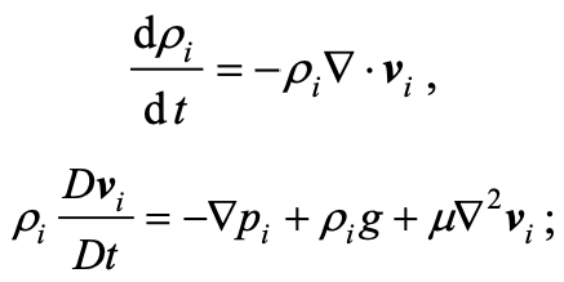SPH方法的核心思想是以离散化粒子的形式来表征连续的场, 并对场量使用积分近似的方式进行计算。位置在xi粒子i的场量：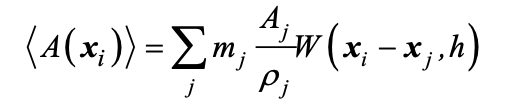第i个粒子的密度计算公式为：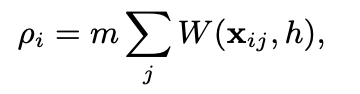压力场直接从Navier-Stokes方程式推导而得：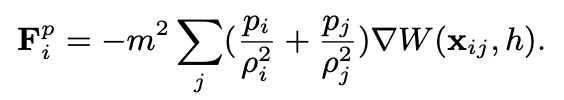PCISPH算法

在PCISPH方法中，速度和位置会及时更新，并估计新的粒子密度。然后，对于每个粒子，计算出参考密度的预测变化，并用于更新压力值，压力值又进入压力的重新计算。此过程一直迭代到收敛，即直到所有粒子密度波动均小于用户定义的阈值η(例如1％)。在完成矫正后，我们再更新速度和位置。详细算法流程图如下：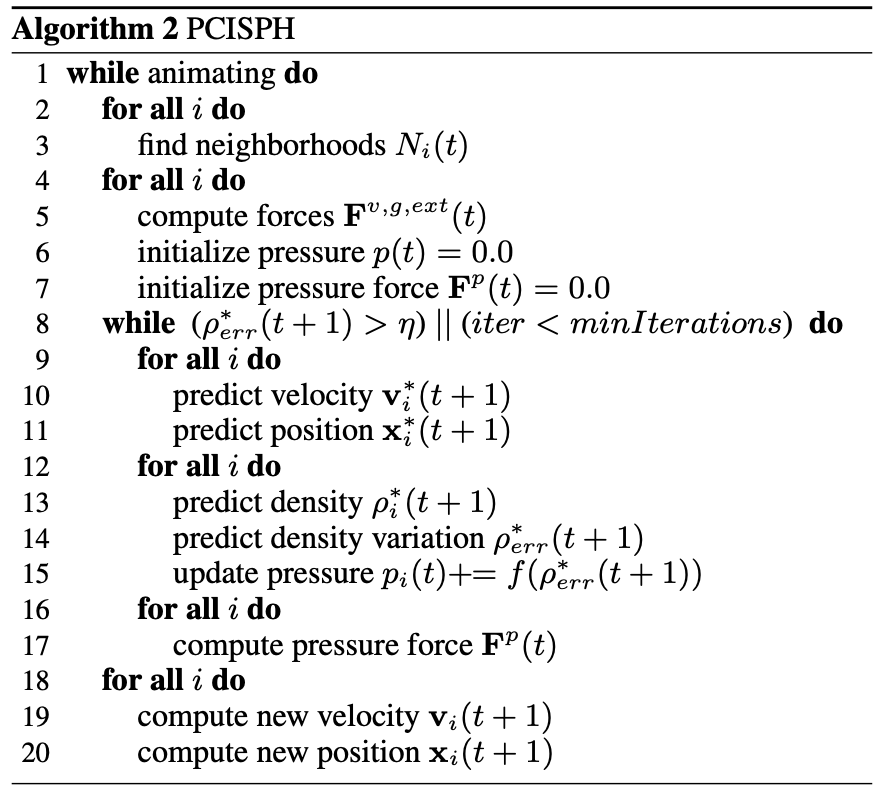该算法总体思路如下：

1.计算每个粒子的邻居信息，并记录在邻接表内。

2.计算出了压力之外的所有其他力(黏力，重力)。

3.执行矫正循环：执行1).，2).，3).。

1).预测所有粒子新的的速度和位置。

2).预测所有粒子新的密度，以及计算新密度和旧密度之间的差值。

3).预测所有粒子的压力。

4.完成矫正后，利用矫正的压力计算粒子速度和位置。

压强导数

我们的算法是根据预测的密度计算新的压力值，因此我们需要找到它们之间变化的关系。我们的目的是找到一个压强p，该压强以这样一种方式改变粒子的位置，即预测的密度与参考密度相对应。

对于给定的内核平滑长度h，使用SPH密度求和公式计算时间点t + 1处的密度：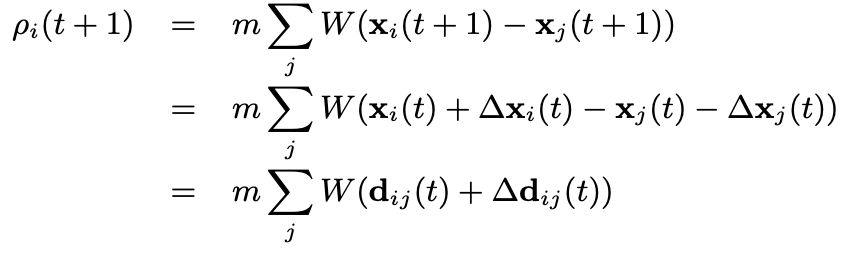其中，。假定非常小，我们可以用一阶泰勒展开近似：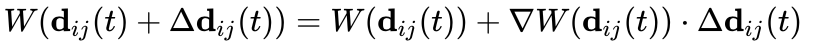带入得：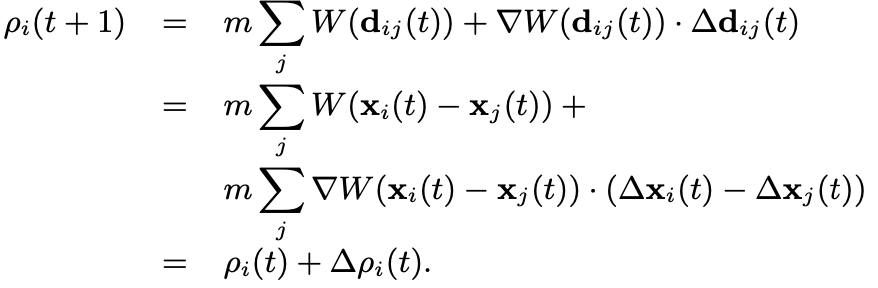我们令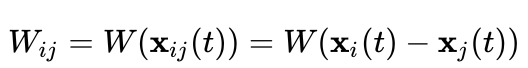，因此密度增量公式为：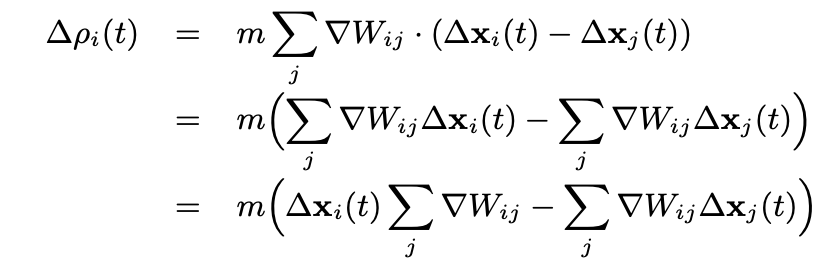我们假设邻居具有相等的压强且密度为静止密度(根据不可压缩性条件)，则结果是：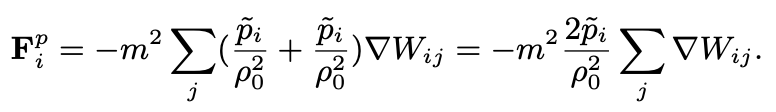PCISPH算法在每次迭代校正时只矫正d流体粒子的压力，通过迭代计算出的压力让粒子之间不至于靠的过近(粒子之间距离太近可以理解为流体可压缩)。在只考虑压力的情况下，根据时间积分可以计算出粒子在该压力作用下的位移：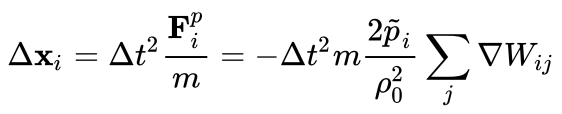粒子i在获得粒子j的压力同时，也会对领居粒子j施加反作用力，因此：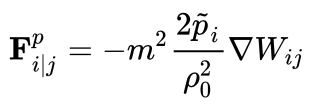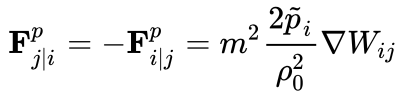同样，由于粒子i导致粒子j产生的位移为：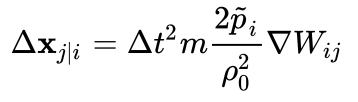将位移增量带入密度增量公式：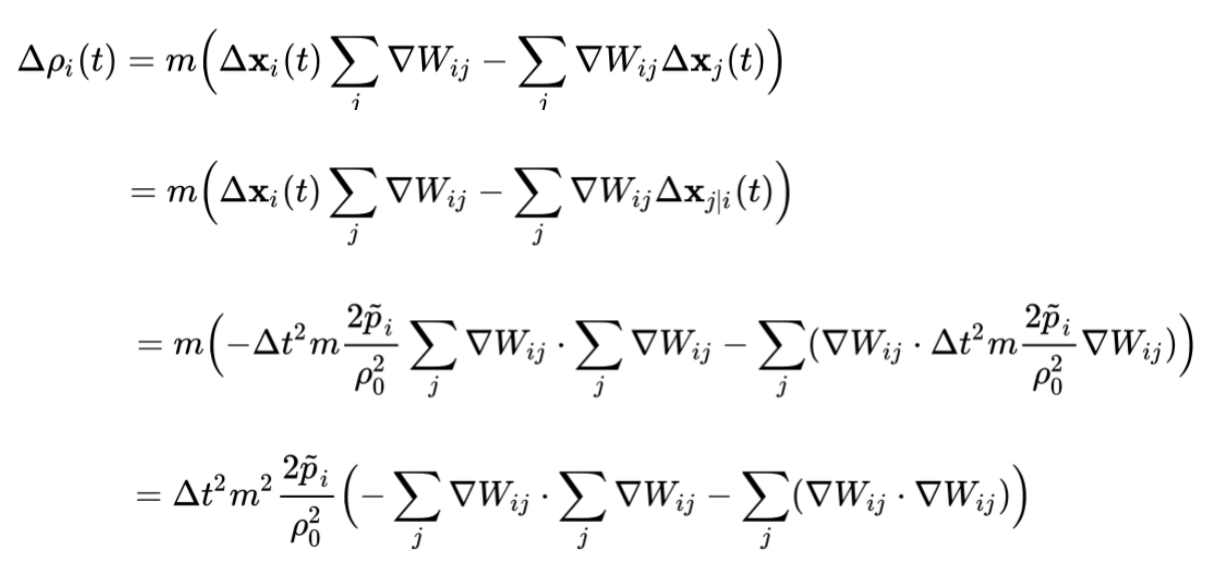因此为：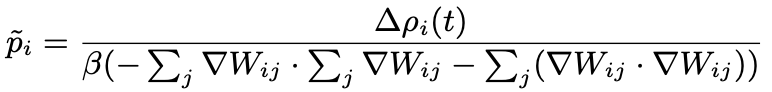其中为：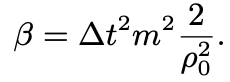公式表示了我们迭代过程中，需要不断变化的密度值，从而满足粒子不可压缩性。而改变的密度需要改变的压强。我们每次预测的粒子密度为：。预测密度和静止密度之间的误差为：。而我们的目标是让粒子密度为，这需要的密度改变量为。因此，我们需要施加的压强值为：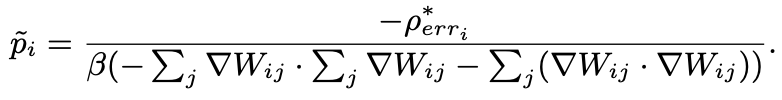这个压强计算公式在邻居粒子数目不足的时候会导致计算错误，解决办法是进行一次预计算，即在流体粒子周围充满邻居粒子的情况下计算。我们可以直接在初始化流体时计算一次系数即可：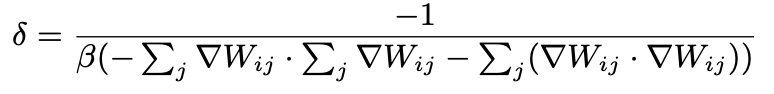因此，我们的压强改变量为：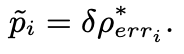由于只要不满足不可压缩条件，我们就重复进行预测校正步骤，因此，我们需要在迭代中不断矫正压强：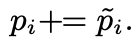算法实现

    void FluidSystem::_init(unsigned int maxPointCounts, const fBox3 &wallBox, const fBox3 &initFluidBox, const glm::vec3 &gravity){
m_pointBuffer.reset(maxPointCounts);
m_sphWallBox=wallBox;
m_gravityDir=gravity;

//根据粒子间距计算粒子质量
m_pointMass=m_restDensity*pow(m_pointDistance,3.0);
//设定流体块

//MarchingCube算法属性
m_mcMesh=rxMCMesh();
m_field=new float[(m_rexSize+1)*(m_rexSize+1)*(m_rexSize+1)]();
posData=std::vector<float>(3*m_pointBuffer.size(),0);
m_hPosW.resize(3*m_pointBuffer.size(),0.0);

m_gridContainer.insertParticles(&m_pointBuffer);//每帧刷新粒子位置
m_neighborTable.reset(m_pointBuffer.size());//重置邻接表

//计算W的梯度
//计算因子
_computeDensityErrorFactor();
}

void FluidSystem::_computeGradWValues(){
const int numP=m_pointBuffer.size();
for (int i=0; i<numP; i++) {
Point *pi=m_pointBuffer.get(i);
}
for (int i=0; i<numP; i++) {
Point *pi=m_pointBuffer.get(i);
pi->kernel_self=_computeNeighbor(i);
m_neighborTable.point_commit();
int neighborCounts=m_neighborTable.getNeighborCounts(i);
//预测密度计算
for (int j=0; j<neighborCounts; j++) {
unsigned int neighborIndex;
float r;
m_neighborTable.getNeighborInfo(i, j, neighborIndex, r);
Point* pj=m_pointBuffer.get(neighborIndex);
//需要用预测的位置去重新计算距离和核值
glm::vec3 pi_pj=(pi->pos-pj->pos)*m_unitScale;
float r2=pi_pj.x*pi_pj.x+pi_pj.y*pi_pj.y+pi_pj.z*pi_pj.z;
if(h2>r2){
float h2_r2=h2-r2;
r=pow(r2,0.5f);

}
}
}
}

我们在其中计算所有粒子的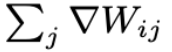和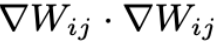。

之后我们在函数_computeDensityErrorFactor()中计算系数，代码如下：

void FluidSystem::_computeDensityErrorFactor(){
int maxNeighborIndex = 0;
int maxNeighs = 0;
const int numParticles = m_pointBuffer.size();
for (int i=0; i<numParticles; i++) {
Point *pi=m_pointBuffer.get(i);
int neighborCounts=m_neighborTable.getNeighborCounts(i);
int numNeighbors=0;
//预测密度计算
for (int j=0; j<neighborCounts; j++) {
unsigned int neighborIndex;
float r;
m_neighborTable.getNeighborInfo(i, j, neighborIndex, r);
Point* pj=m_pointBuffer.get(neighborIndex);
//需要用预测的位置去重新计算距离和核值
glm::vec3 pi_pj=(pi->pos-pj->pos)*m_unitScale;
float r2=pi_pj.x*pi_pj.x+pi_pj.y*pi_pj.y+pi_pj.z*pi_pj.z;
if(h2>r2){
numNeighbors++;
}
}
//获取邻居最多的粒子，和邻居个数
if (numNeighbors>maxNeighs) {
maxNeighs=numNeighbors;
maxNeighborIndex=i;
}
}
//获取邻居最多的粒子
Point* pmax=m_pointBuffer.get(maxNeighborIndex);

//计算新的压力根据密度误差
float restVol=m_pointMass/m_restDensity;
float preFactor=2.0*restVol*restVol*m_deltaTime*m_deltaTime;
m_densityErrorFactor=-1.0/divisor;
const float factor = m_deltaTime / 0.0001f;
float densityErrorFactorParameter = 0.05 * factor * factor;
m_densityErrorFactor*=1.0*densityErrorFactorParameter;

}

值得注意的是，我们还在系数之前乘上densityErrorFactorParameter影响系数，该系数受影响。如果不乘上该系数就会出错，论文里并没有明确提出。

计算完成预计算的系数后，我们的tick()函数如下：

    void FluidSystem::tick(bool suf){
//求解领居结构
m_gridContainer.insertParticles(&m_pointBuffer);//每帧刷新粒子位置
m_neighborTable.reset(m_pointBuffer.size());//重置邻接表

//计算外力
_computerExternalForces();
//预测矫正
_predictionCorrection();
//更新速度和位置
_updatePosAndVel();

//用于构建表面
glm::vec3 tem=m_sphWallBox.min-glm::vec3(1.0);
if(suf){
CalImplicitFieldDevice(m_rexSize, tem, glm::vec3((1.0/m_gridScale)/m_gridContainer.getDelta()), m_field);
clearSuf();//清空表面数据
m_mcMesh.CreateMeshV(m_field, tem, (1.0/m_gridScale)/m_gridContainer.getDelta(), m_rexSize, m_thre, m_vrts, m_nrms, m_face);
}
}

我们按照算法流程，首先求解领居结构，这在之前的章节里介绍过，这里就不多提了。

然后计算除了压力的所有外力，该函数_computerExternalForces()代码如下：

    void FluidSystem::_computerExternalForces(){
for(int i=0;i<m_pointBuffer.size();i++){
Point* pi=m_pointBuffer.get(i);
pi->forces=glm::vec3(0.0);

//邻居粒子装载
pi->kernel_self=_computeNeighbor(i);
m_neighborTable.point_commit();

//外力计算
int neighborCounts=m_neighborTable.getNeighborCounts(i);
const float restVolume=m_pointMass/m_restDensity;
for(unsigned int j=0;j<neighborCounts;j++){
unsigned int neighborIndex;
float r;
m_neighborTable.getNeighborInfo(i, j, neighborIndex, r);
Point* pj=m_pointBuffer.get(neighborIndex);

//F_Viscosity
//m_kernelViscosity = 45.0f/(3.141592f * h^6);
float vterm=m_kernelViscosity*m_viscosity*h_r*restVolume*restVolume;
pi->forces-=(pi->velocity-pj->velocity)*vterm;
}
//F_gravity
pi->forces+=m_gravityDir*m_pointMass;

//F_boundary
pi->forces+=_boundaryForce(pi)*m_pointMass;

//初始化矫正因子
pi->correction_pressure=0.0f;
pi->correction_pressure_froce=glm::vec3(0.0);
}
}

计算完成外力后，进入矫正预测环节，该函数_predictionCorrection()代码为：

void FluidSystem::_predictionCorrection(){
_density_error_too_large=true;
int iteration=0;
while ((iteration<minLoops)||((_density_error_too_large)&&(iteration<maxLoops))) {
for(int i=0;i<m_pointBuffer.size();i++){
Point* p=m_pointBuffer.get(i);
_predictPositionAndVelocity(p);
}

//循环终止条件(所有粒子的最大预测密度)
_max_predicted_density=0.0;

for(int i=0;i<m_pointBuffer.size();i++){
_computePredictedDensityAndPressure(i);
}

//循环终止条件
float densityErrorInPercent=max(0.1f*_max_predicted_density-100.0f,0.0f);
float maxDensityErrorAllowed=1.0f;

//如果密度误差小于终止条件，则终止循环(小于密度误差阈值)
if(densityErrorInPercent<maxDensityErrorAllowed)
_density_error_too_large=false;

for (int i=0; i<m_pointBuffer.size(); i++) {
_computeCorrectivePressureForce(i);
}
iteration++;
}
}

其中我们设置最小循环次数为3，最大循环次数为50。在矫正过程中，我们继续细分为3个步骤：

1).预测所有粒子的速度和位置，该函数_predictPositionAndVelocity(p)代码为：

void FluidSystem::_predictPositionAndVelocity(Point* p){
// v' = v + delta_t * a
// a = F / m
// v' = v + delta_t * F * V / m
// v' = v + delta_t * F * 1/density

//计算预测速度和位置
p->predicted_velocity=p->velocity+(p->forces+p->correction_pressure_froce)*m_deltaTime/m_pointMass;
p->predicted_position=p->pos+p->predicted_velocity*m_deltaTime;

//碰撞处理
_collisionHandling(p->predicted_position,p->predicted_velocity);

//初始化预测密度
p->predicted_density=0.0;
}

值得注意的是，因为是预测的速度，所以需要好用矫正后的压力去计算。

2).计算预测的密度和压力，该函数_computePredictedDensityAndPressure(i)的代码为：

void FluidSystem::_computePredictedDensityAndPressure(int i){
Point* pi=m_pointBuffer.get(i);
int neighborCounts=m_neighborTable.getNeighborCounts(i);
pi->predicted_density=pi->kernel_self*m_pointMass;
//预测密度计算
for (int j=0; j<neighborCounts; j++) {
unsigned int neighborIndex;
float r;
m_neighborTable.getNeighborInfo(i, j, neighborIndex, r);
Point* pj=m_pointBuffer.get(neighborIndex);
//需要用预测的位置去重新计算距离和核值
glm::vec3 pi_pj=(pi->predicted_position-pj->predicted_position)*m_unitScale;
float r2=pi_pj.x*pi_pj.x+pi_pj.y*pi_pj.y+pi_pj.z*pi_pj.z;
if(h2>r2){
float h2_r2=h2-r2;
pi->predicted_density+=m_kernelPoly6*pow(h2_r2, 3.0)*m_pointMass;
}
}
auto sss=pi->predicted_density;
//计算密度误差，仅考虑正向误差
pi->density_error=max(pi->predicted_density-m_restDensity,0.0f);

//更新压力,只矫正正压力，densityErrorFactor被预先计算并用作常数
pi->correction_pressure+=max(pi->density_error*m_densityErrorFactor,0.0f);

_max_predicted_density=max(_max_predicted_density,pi->predicted_density);

}

值得注意的是，我们这里都只考虑正向的误差，即压缩的密度误差和压强误差，拉伸的我们不做考虑。

3).计算矫正后的压力。该函数_computeCorrectivePressureForce(i)代码为：

void FluidSystem::_computeCorrectivePressureForce(int i){
Point* pi=m_pointBuffer.get(i);
int neighborCounts=m_neighborTable.getNeighborCounts(i);
pi->correction_pressure_froce=glm::vec3(0.0f);

float densSq=m_restDensity*m_restDensity;
float pi_pres=pi->correction_pressure;

//更新压力
for (int j=0; j<neighborCounts; j++) {
unsigned int neighborIndex;
float r;
m_neighborTable.getNeighborInfo(i, j, neighborIndex, r);
Point* pj=m_pointBuffer.get(neighborIndex);
//需要用预测的位置去重新计算距离和核值
glm::vec3 pi_pj=(pi->pos-pj->pos)*m_unitScale;
float r2=pi_pj.x*pi_pj.x+pi_pj.y*pi_pj.y+pi_pj.z*pi_pj.z;
if(h2>r2){
r=pow(r2,0.5);
float pj_pres=pj->correction_pressure;
}
}
}

这里，我们的预测矫正迭代代码就结束了，我们推出迭代后，利用矫正好的压力求解新的速度和位置，该函数_updatePosAndVel()代码如下：

void FluidSystem::_updatePosAndVel(){
for(int i=0;i<m_pointBuffer.size();i++){
Point* pi=m_pointBuffer.get(i);
pi->forces+=pi->correction_pressure_froce;
const float invMass=1.0/m_pointMass;

//        //Symplectic Euler Integration
//        pi->velocity+=pi->forces*invMass*m_deltaTime;
//        pi->pos+=pi->velocity*m_deltaTime/m_unitScale;

//Leapfrog integration
glm::vec3 vnext = pi->velocity + pi->forces*invMass*m_deltaTime; // v(t+1/2) = v(t-1/2) + a(t) dt
pi->velocity_eval = (pi->velocity + vnext)*0.5f;  //v(t+1) = [v(t-1/2) + v(t+1/2)] * 0.5
pi->velocity = vnext;
pi->pos += vnext*m_deltaTime/m_unitScale;  // p(t+1) = p(t) + v(t+1/2) dt

//弹入位置数据
posData[3*i]=pi->pos.x;
posData[3*i+1]=pi->pos.y;
posData[3*i+2]=pi->pos.z;

}
}

完成结果图：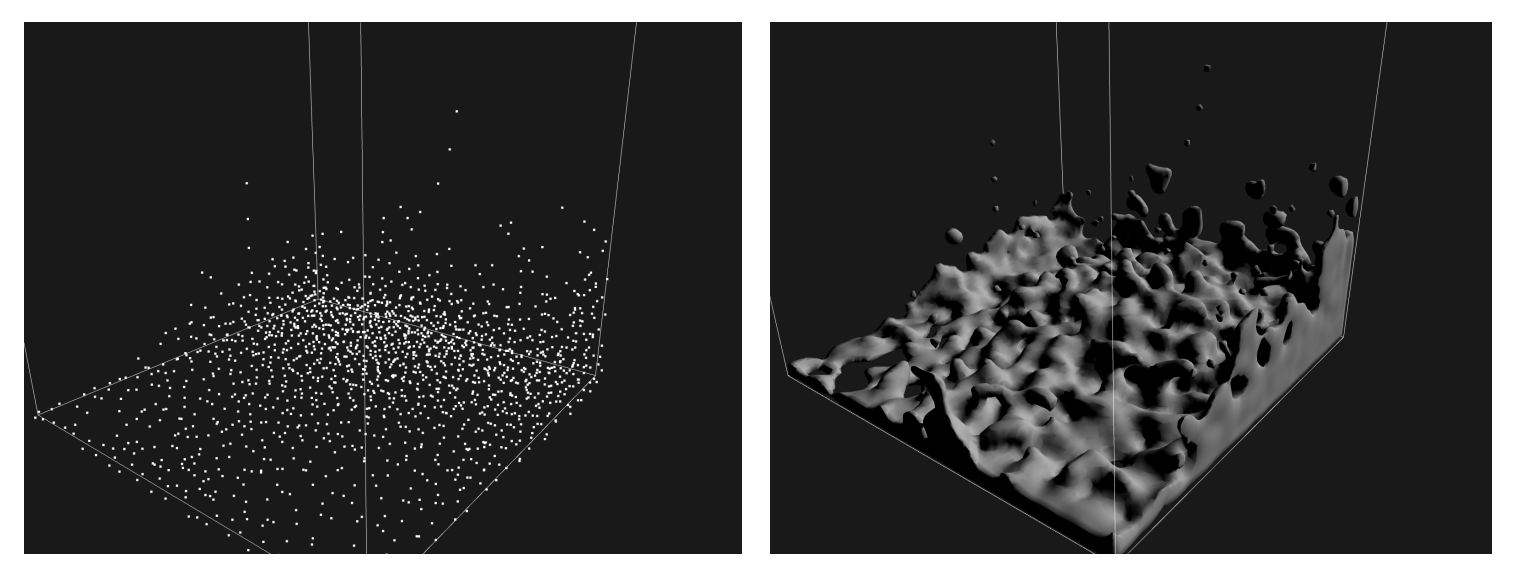对比普通SPH，我们发现在挤压处，PCISPH有明显的矫正。普通SPH效果如下：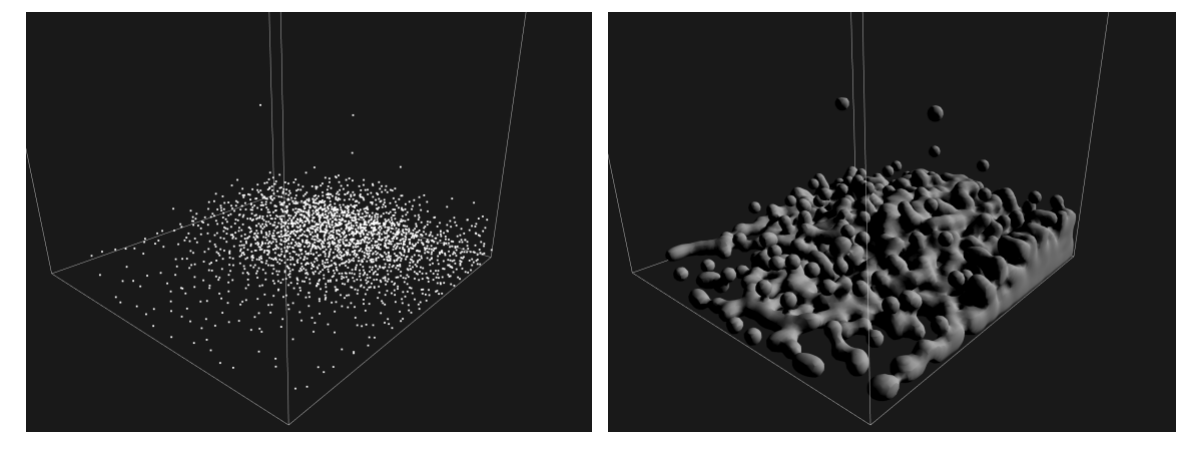展开全文• 今天我们就从最简单的自由落体运动开始，来对MATLAB仿真作一个初步的认识。 我们这次要分析的自由落体运动情景为： 一个可视为质点的小球从离地面45米高度处自由下落，空气阻力忽略，重力加速度为10m/s^2. 以上就是...
• 模拟流体在三边固定且顶边（盖子）在水平方向上均匀移动的方形空腔中的运动。 流函数涡度公式用于对问题进行建模。 有限差分法用于离散控制偏微分方程。 用户可以输入雷诺数、边长、空间网格点数、时间步长和结束...
• 该代码模拟了N个聚苯乙烯球体在密闭... 第一个代码是所有运动发生的地方，球体在每一步的位置都被保存，第二个代码为球体的运动设置动画，第三个代码使用亥姆霍兹定理计算盒子每个点的速度近似于无旋和不可压缩流体
• matlab四杆机构运动仿真代码机器自动化中的建模和实时仿真 本练习的目的是建立4执行器自由度（DOF）液压装载机的仿真模型（如下图所示），以在实时计算机中对其进行仿真。 1.简介 建立了四执行器自由度（DOF）液压...
• ## 基于D3Q19模型的LBM流体模拟

万次阅读 热门讨论 2009-05-14 00:06:00
在CUDA上实现基于D3Q19模型的LBM流体模拟方法简介 --cyrosly与2009.05.14下午   LBM（Lattice Boltzmann Model）方法简介：  LBM是始与上世纪80年代末起源于70年代的LGA（格子气自动机）理论模型，并最终从...cuda thread 网格 float
• 洛伦兹是世界上第一个从确定的方程中发现了非周期的混沌现象。洛伦兹方程是描述空气流体运动的一个简化微分方程组。本文提供了洛伦兹方程的MATLAB实现
• 该技术允许以亚毫米级的精度和亚毫秒级的时间分辨率跟踪粒子或流体运动，并且由于使用了高穿透性的511keV伽马射线，因此可以用于探测更大，密集，光学上的内部动力学不透明的系统-非常适合工业和科学应用。...
• Eshelby张量数值评估的最佳方案及其在MATLAB软件包中的实现，用于模拟粘性流体在缓慢流动中的运动孟梦萌，姜大志，露西X.卢计算机与地球科学，第96卷，2016年11月，第98-108页 使用此或衍生代码生成图形或其他内容的...
• ## matlab之产品大全

千次阅读 多人点赞 2020-12-16 19:30:59
matlab大全链接：入口1或者入口2 这里仅对matlab存在的工具做一个简单梳理，方便日后使用。 Matlab版本：R2020b 应用如下： 一、数学、统计和优化 1.曲线拟合工具箱 使用回归，插值和平滑使曲线和曲面适合数据...
• 孤子这个名词首先是在物理的流体力学中提出来的。1834年在爱丁堡到格拉斯哥的运河上，苏格兰海军工程师罗素(J.Scott Russell)观察到一种奇特的水波，并在1844年发表的一份报告中描述了这种奇特水波，并称这种波为...
• 湍流、复杂流动、非定常流动等现象一直是流体力学中重要的研究对象及疑难问题，因此开发适于流体运动研究的方法与技术也始终是一个重要的课题。早期发明的热线热膜流速计（HWFA）至今已有80多年的历史，曾经为流动...大数据 机器学习 java python 人工智能
• 下载&nbsp;&gt;&nbsp; 信息化&nbsp;&gt;&nbsp; 其它&nbsp;&gt;&nbsp;基于蒙特卡罗法的二维随机裂隙模拟Matlab程序 ...
• 在我的博士学位中，我研究了浸没在流体中并受到外部旋转磁场作用的顺磁性粒子的短链。 这些粒子系统在微流控，光学和生物行业中具有许多成熟的潜在应用。 上传的论文是我的第一篇博士学位论文，讨论了我最重要的博士...
• （附加质量实际上就是说在水里物体运动要带动周围流体一块运动，这种效应可以近似看做为本体的质量增加了，增加的这部分质量就是附加质量。） 那么这样子是否真的可以产生推力呢？ 直觉不可靠，让我们从一个简单...
• 使用Buongiorno的纳米流体模型对纳米流体进行了模拟，并且将边界上的纳米流体颗粒分数视为被动而非主动控制。 将非相似解应用于控制方程，并使用MATLAB函数bvp4c求解所得系统。 研究了滑移参数，比奥数，辐射参数，...
• 文章目录一、动态系统1、动态系统所用数学模型2、连续系统建模3、离散系统建模4、线形系统5、线性离散系统 一、动态系统 1、动态系统所用数学模型 常微分方程 求解方法：欧拉法、龙格-库塔法及其改进解法、Adams外......

# matlab模拟流体运动matlab 订阅